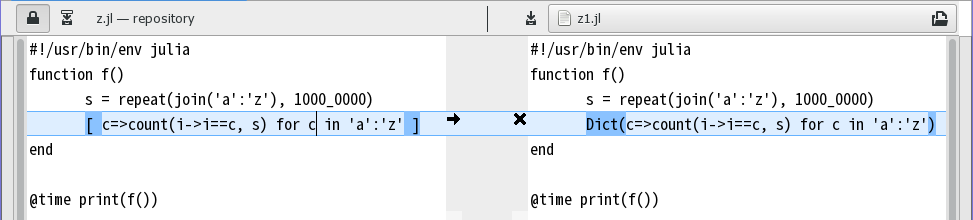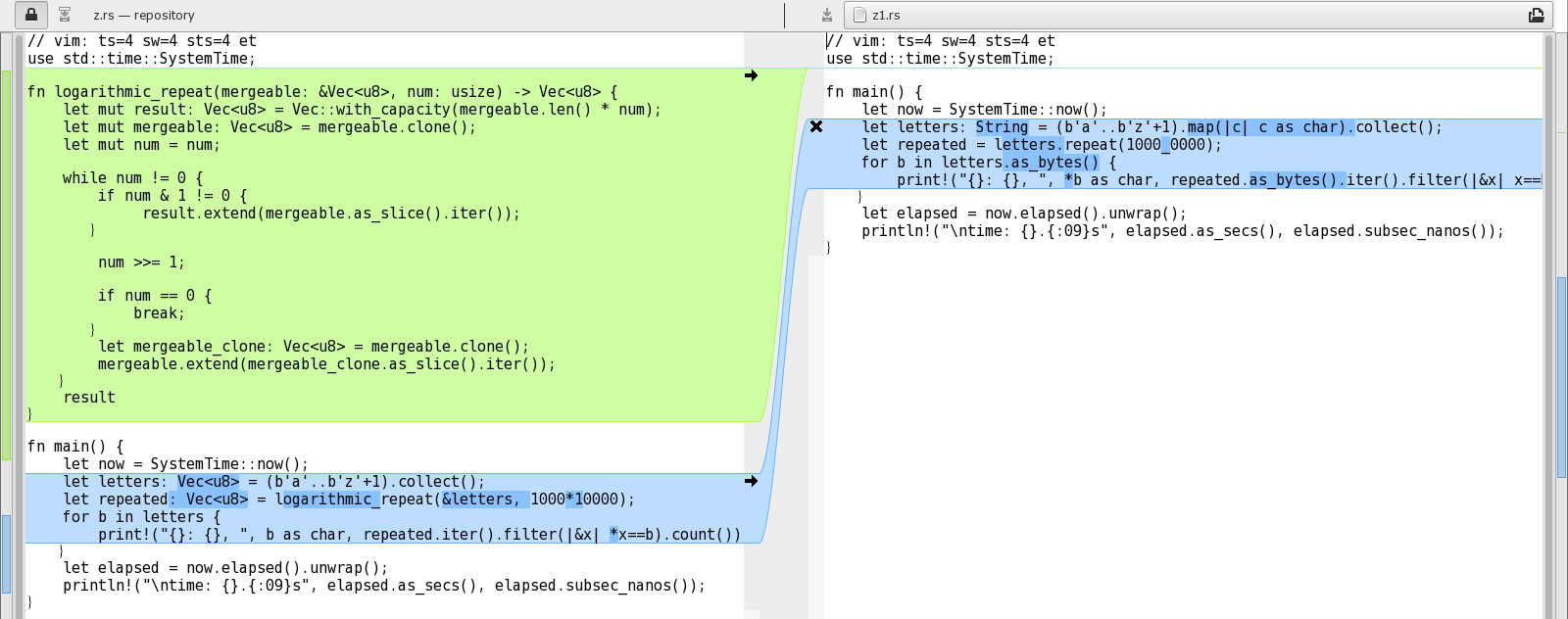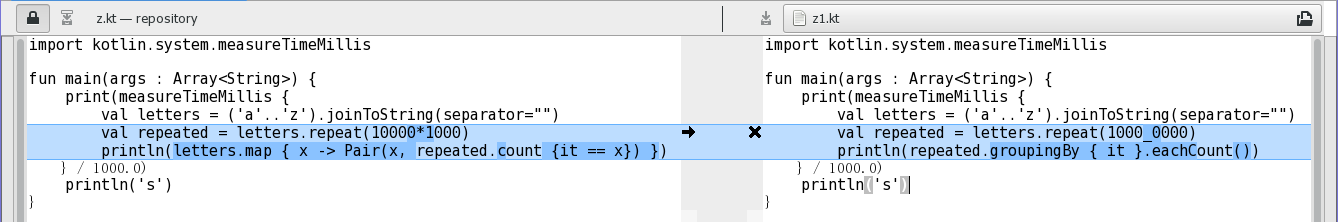【来做题】功能实现倒是很简单~ 用你熟悉的语言，统计一个字符串abcdefghijklmnopqrstuvwxyz…abcdefghijklmnopqrstuvwxyz（1千万个a-z，不可直接a=1千万……） 中每个字母的个数，最后输出类似图示。要求除了更好的方式（如更加Pythonic的方式），还要计算越快越好，并打印出代码执行时间（打印效果类似图示）AWS c4.xlarge `1.142s` `2.559s` `3.018s` `5.148s` `9.013s` `17.515s`

Julia

``````function f()
s = repeat(join('a':'z'), 1000_0000)
Dict(c=>count(i->i==c, s) for c in 'a':'z')
end

@time print(f())
``````Rust

Rust 版代码变化很大。上篇中提到为了实现对数级重复写了不少冤枉代码，现在不用了，因为 Rust 在 1.16 起（这次用的是 1.17）标准库就自带字符串重复了，这一版用的代码如下：

``````// 编译方式 rustc -C opt-level=3 z1.rs
use std::time::SystemTime;

fn main() {
let now = SystemTime::now();
let letters: String = (b'a'..b'z'+1).map(|c| c as char).collect();
let repeated = letters.repeat(1000_0000);
for b in letters.as_bytes() {
print!("{}: {}, ", *b as char, repeated.as_bytes().iter().filter(|&x| x==b).count());
}
let elapsed = now.elapsed().unwrap();
println!("\ntime: {}.{:09}s", elapsed.as_secs(), elapsed.subsec_nanos());
}
``````Java 8

Java 版代码无变化，新的 Java 稳定版没有本质改动。但由于其他语言的进步，上次最快的代码如今在速度上已经平淡无奇了。

Kotlin

``````import kotlin.system.measureTimeMillis

fun main(args : Array<String>) {
print(measureTimeMillis {
val letters = ('a'..'z').joinToString(separator="")
val repeated = letters.repeat(1000_0000)
println(repeated.groupingBy { it }.eachCount())
} / 1000.0)
println('s')
}
``````Swift

``````// 编译方式 swiftc -O y1.swift
import Foundation

let date = Date()

let letters = (0..<26).map({String(UnicodeScalar(UnicodeScalar("a").value + \$0)!)}).joined()
let repeated = String(repeating: letters, count: 1000_0000)

for c in letters.utf8 {
print(Character(UnicodeScalar(c)), repeated.utf8.filter{\$0 == c}.count, separator:": ", terminator:", ")
}

let elapseTimeInSeconds = Date().timeIntervalSince(date as Date)
print()
print(elapseTimeInSeconds)
``````

小结

• Julia 的性能提升了一个数量级。
• Rust 支持高效字符串重复。
• Kotlin 支持 `groupingBy``eachCount` 以及数字字面值分隔符。